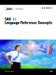# Constructing Efficient WHERE Expressions

Indexing a SAS data set can significantly improve the performance of WHERE processing. An index is an optional file that you can create for SAS data files in order to provide direct access to specific observations. Processing a WHERE expression without an index requires SAS to sequentially read every observation to find the ones that match the selection criteria. Having an index allows the software to determine which observations satisfy the criteria without having to read all the observations, which is referred to as optimizing the WHERE expression. However, by default, SAS decides whether to use the index or read the entire data set sequentially. For details on how SAS uses an index to process a WHERE expression, see 'Using an Index for WHERE Processing' on page 527.

In addition to creating indexes for the data set, here are some guidelines for writing efficient WHERE expressions:

Table 12.4: Constructing Efficient WHERE Expressions

Guideline

Efficient

Inefficient

Avoid using the LIKE operator that begins with % or _.

where country like 'A%INA';

where country like '%INA';

Avoid using arithmetic expressions.

where salary > 48000;

where salary > 12*4000;

Use the IN operator instead of a compound expression.

where state in ('NC' , 'PA' , 'VA');

where state ='NC' or state = 'PA' or state = 'VA';SAS 9.1 Language Reference Concepts
ISBN: 1590471989
EAN: 2147483647
Year: 2004
Pages: 255